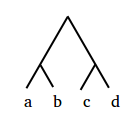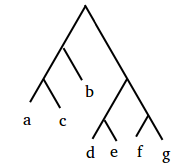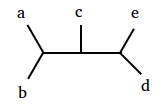# Glossary

## Newick format

Newick format offers a way of representing binary trees in text form by using parentheses and commas. Here's an example of a straightforward rooted tree.According to this tree, taxa $a$ and $b$ appear to be closely related, as do $c$ and $d$. More formally, $a$ and $b$ are said to be neighbors since they are adjacent to the same internal node of the tree. We label this internal node using the syntax $(a, b)$, then delete $a$ and $b$ and iterate the process. In the end, the above tree can be represented using Newick format as $((a,b),(c,d))$.The above rooted tree, which is more complex, can be represented by the following steps:

• consolidate $a$ and $c$ into $(a, c)$;
• consolidate $(a, c)$ and $b$ into $((a, c), b)$;
• consolidate $d$ and $e$ into $(d, e)$;
• consolidate $f$ and $g$ into $(f, g)$;
• consolidate $(d, e)$ and $(f, g)$ into $((d, e), (f, g))$;
• consolidate the final two leaf nodes, $((a, c), b)$ and $((d, e), (f, g))$, into the tree's final Newick representation $(((a, c), b), ((d, e), (f, g)))$.For unrooted trees, we may arbitrarily construct a root on any one of the tree's edges. root picked arbitrarily at one of the internal edges. As a result, we will wind up with different possible Newick formats. For example, the tree above tree can be represented as $(((a,b),c),(d,e))$ or $((a,b),(c,(d,e)))$; try to find another representation of it.